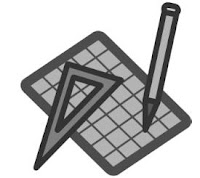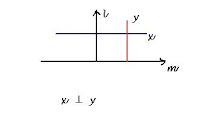## Tuesday, 14 August 2012

### CBSE - Class 9 - Maths - Lines and Angles (Ex. 6.2)

Lines and Angles
(NCERT Ex. 6.2 and Q & A from CBSE papers)1. Corresponding angles axiom: If a transversal intersects two parallel lines, then each pair of corresponding angles is equal.

2. If a transversal intersects two lines such that a pair of corresponding angles is equal, then the two lines are parallel to each other.

3. If a transversal intersects two parallel lines, then each pair of alternate interior angles is equal.

4. If a transversal intersects two lines such that a pair of alternate interior angles is equal, then the two lines are parallel.

5. If a transversal intersects two parallel lines, then each pair of interior angles on the same side of the transversal is supplementary.

6. If a transversal intersects two lines such that a pair of interior angles on the same side of the transversal is supplementary, then the two lines are parallel.

7. Lines which are parallel to the same line are parallel to each other.

Exercise 6.2

Q1: In Fig. below, find the values of x and y and then show that AB || CD.
Answer:   ∠50° + x = 180°              (Linear pair)
⇒ x = 180° - 50° = 130°

Since 130 and y are vertically opposite angles and hence are equal
⇒ y = 130°

Since x and y angles are equal and form alternate interior angles of line AB and CD,
⇒ AB || CD

Q2: In Figure, if AB || CD, CD || EF and y : z = 3 : 7, find x.

Answer: Lines which are parallel to the same line are parallel to each other.
Since  AB || CD and CD || EF.
∴ AB || EF.
and  x = z                   (alternate interior angles)

Given, y:z = 3:7
⇒ y = 3k and z = 7k where k is a constant.               .... (I)

Since a pair of interior angles on the same side of the transversal is supplementary.
∴ x + y = 180°
z + y = 180°

From eq. I,

7k + 3k = 180°
10k = 180°
⇒ k = 18°
⇒ y = 3 × 18° = 54°
and x = z = 7 × 18° = 126°

Q3: In Figure below, if AB || CD, EF ⊥ CD and ∠GED = 126°, find ∠AGE, ∠GEF and ∠FGE.

Answer: Given,  ∠GED = 126°
also  ∠GED = ∠GEF + ∠FED

⇒ ∠GEF + ∠FED =  126°
⇒ ∠GEF + 90° = 126°                        ( EF ⊥ CD ∴ ∠FED = 90°)
⇒ ∠GEF = 126° - 90° = 36°

Since AB || CD,  ∠AGE and ∠GED form alternate co-interior angles and are equal.
i.e. ∠AGE = ∠GED = 126°

∠AGE and ∠FGE form a linear pair.
⇒ ∠AGE + ∠FGE = 180°
⇒ ∠FGE = 180° -  ∠AGE = 180° - 126°
⇒ ∠FGE = 54°

Q4: In Figure below, if PQ || ST, ∠PQR = 110° and ∠RST = 130°, find ∠QRS.
[Hint : Draw a line parallel to ST through point R.]

Answer:  Draw a line OL parallel to ST passing through point R.
Since PQ || ST and ST || OL  ⇒ PQ || OL
∠PQR and ∠QRO are interior angles on same side of transversal QR.
∴ ∠PQR + ∠QRO = 180°
⇒ ∠QRO = 180° -  ∠PQR = 180° - 110° = 70°

∠RST and ∠SRL are interior angles on same side of transversal RS.
∴ ∠RST + ∠SRL = 180°
⇒ ∠SRL = 180° -  ∠RST = 180° - 130° = 50°

OL is a straight line and QR and SR are standing on it.
⇒ ∠QRO + ∠QRS + ∠RST = 180°
⇒ ∠QRS = 180° - (∠QRO + ∠RST) = 180° - ( 70° + 50°) = 180° - 120°=  60°

Q5: In Fig. below, if AB || CD, ∠ APQ = 50° and ∠ PRD = 127°, find x and y.

Answer:  Since AB || CD
∠APQ = ∠PQR          (Alternate interior angles of transversal PQ)
⇒ 50° = x
or x = 50°

∠APR = ∠PRD       (Alternate interior angles of transversal PR)
⇒ 50° + y =  127°
⇒ y = 127° - 50° = 77°

Q6: In figure below, PQ and RS are two mirrors placed parallel to each other. An incident ray AB strikes the mirror PQ at B, the reflected ray moves along the path BC and strikes the mirror RS at C and again reflects back along CD. Prove that AB || CD.

Answer: Draw perpendiculars BL and CM such that BL ⊥ PQ and CM ⊥ RS
According to laws of reflection, ∠of incidence = ∠ of reflection.
⇒ ∠1 = ∠2                                                   (I)
and ∠3 = ∠4                                                 (II)

∵ PQ || RS                                                     (Given)
and BL ⊥ PQ and CM ⊥ RS                       (By construction)

⇒BL || CM
⇒ ∠2 = ∠3                                                    (alternate angles)
⇒ ∠1 = ∠2 = ∠3 = ∠4                                   (from eq. I and II)

⇒ ∠1 + ∠2 = ∠3 + ∠4
⇒ ∠ABC = ∠BCD
Since  ∠ABC and ∠BCD form pair of alternate angles and are equal.
⇒ AB || CD

Questions from CBSE Examination Papers

Q7(CBSE 2010):  In figure below, AB || CD then find the value of x.
Answer: Given, AB || CD ,  ∠BPQ = 5x - 20°  and  ∠PQD = 2x - 10°

Since  ∠BPQ and ∠PQD of transversal EF are pair of interior angles on the same side. They are supplementary i.e.
∠BPQ + ∠PQD = 180°
⇒  5x - 20° + 2x - 10° = 180°
⇒ 7x - 30° = 180°
⇒ 7x = 180° + 30° =  210°
⇒ x = 210°/7 = 30°Q8(CBSE 2010): Two lines are respectively perpendicular to two perpendicular lines. Then these two lines to each other are:
(a) parallel
(b) perpendicular
(c) inclined at same acute angle
(d) intersecting at 110°

Answer: (b) perpendicular
Note: Question's language is tricky. What it says there are two lines (say l and m) which are perpendicular to each other. If we draw perpendiculars to l and m (say x and y). What shall be x and y?

Q9(CBSE 2011): In fig. below, AB || CD and t is transversal, the value of x is equal to:

(A) 50°
(B) 70°
(C) 35°
(D) 20°

Answer:  ∠(2x + 40)° and ∠(x + 90)° are alternate interior angles and are equal.
(2x + 40)°  = (x + 90)°
2x + 40° = x + 90°
2x -x = 90° - 40°
x = 50°    ...... option (A)#### 1 comment:

1.A linear pair is formed when two adjacent angles have their non-common side in opposite rays. Their measures will always add up to 180 degrees.linear pairs of angles

We love to hear your thoughts about this post!